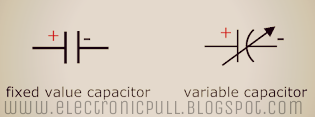# Variable Capacitors - Introduction and Symbol-Titik Letak-Titik Letak

Variable Capacitors:

Variable capacitors are the type of capacitors in which the capacitance can be changed either mechanically or electronically. These type of capacitors are generally used in resonance circuits (LC circuits) in tuning applications and matching of impedance in antennas. These capacitors are known as Tuning Capacitors. There are also other type of variable capacitors which are called Trimmer Capacitor, which are fixed on PCB’s and used to maximize the calibration of devices and equipments. These type of capacitors are small-size-non-polarized capacitors. There value of capacitance is very small,  usually in picofarads (usually less than 500pF).Variable and Fixed Capacitor Symbols

Mechanical variable capacitors includes a set of semi-circular metal plates fixed on the rotor's axis. This setup is placed between a set includes stator metal plates. The whole capacitance value for this capacitor is determined according to the placement of the moving plates of metal corresponding to the fixed metal plates. When the axis is turned, the whole area which is overlapping between the stator and rotor plates will vary and the capacitance changes in it. In this design, where the two sets of plates of metal are fully meshed together , the capacitance value is usually at high value. High voltage type tuning capacitors have large number of air-gap or space between two plates with larger break down voltages in kilo volts. For this large break down voltages, these capacitors are used in tuning circuits.

Mechanical variable capacitors are the type of variable capacitors which uses the air or plastic foils as a dielectric. But the daily use of vacuum variable capacitors is increasing because the working voltage range and capabilities of handling large amount of current is better in these type of capacitors. The capacitance of the mechanically tuned capacitors varies by moving the screw on the top side of it. Where, in electronically controlled variable capacitors, a diode is used in reverse biased condition in which the thickness of the depletion region varies according to the input applied DC voltage. These type of diodes are Variable Capacitance Diodes or Varactors.Variable Capacitor